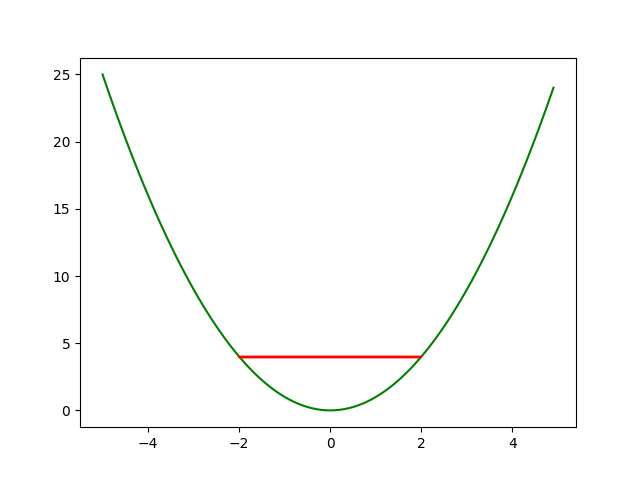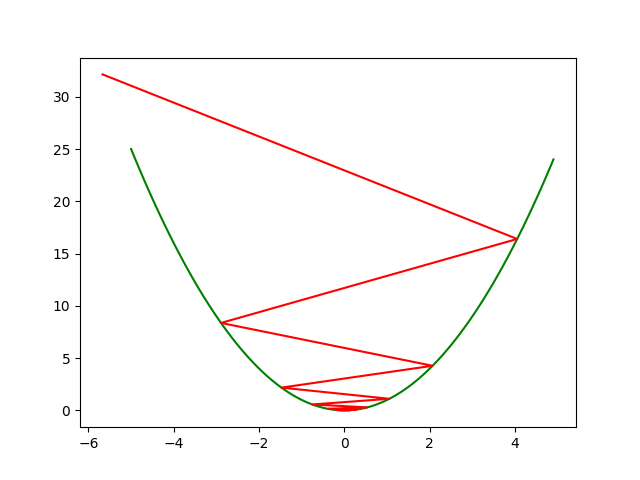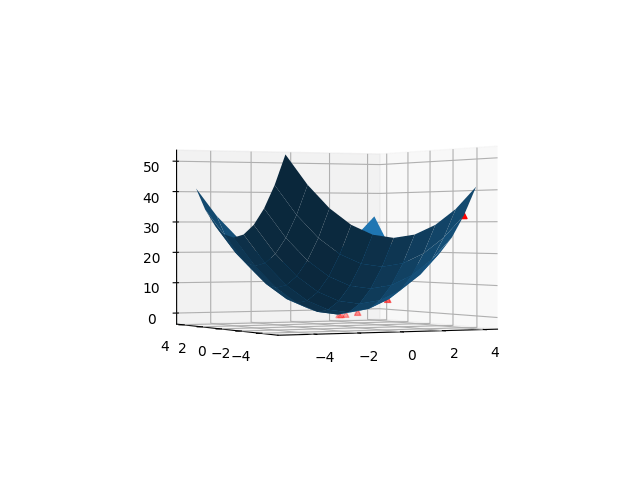``````import numpy as np
x=np.arange(-5,5,0.1) #定义域-5~5
y=x**2 #求解的函数
pointx=[] #用来储存每次梯度下降后的点
x0=-2 #初始值的横坐标-2，随便选的
for i in range(10): #先执行10次
xnew=x0-2*x0 #该点减去该点的导数值
x0=xnew #移动到新的点
pointx.append(x0) #储存点运动轨迹
pointy=np.array(pointx)**2
plt.plot(x,y,color="green") #画函数图像
plt.plot(pointx,pointy,color="red") #画梯度轨迹
plt.show()
````````````import matplotlib.pyplot as plt
ax=plt.axes(projection='3d')
x=np.arange(-5,5)
y=np.arange(-5,5)
X,Y=np.meshgrid(x,y)
Z=X**2+Y**2
ax.plot_surface(X,Y,Z,cmap="rainbow")
plt.show()
````````````import matplotlib.pyplot as plt
x0=4;y0=-4 #初始点
xlist=[x0] #储存x变化
ylist=[y0] #储存y点变化
zlist=[x0**2+y0**2]
alpha=0.3 #学习率0.3
for i in range(5): #执行5次
xnew=x0-2*x0*alpha
ynew=y0-2*y0*alpha
x0=xnew;y0=ynew
xlist.append(x0)
ylist.append(y0)
zlist.append(x0**2+y0**2)
ax.scatter(xlist,ylist,zlist,color='red',marker='^') #画点的位置
plt.show()
``````